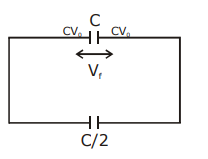# A capacitor C is fully charged with voltage V0.

A capacitor C is fully charged with voltage V0. After disconnecting the voltage source, it is connected in parallel with another uncharged capacitor of capacitance C/2. The energy loss in the process after the charge is distributed between the two capacitors is:

1) $$\frac{1}{2}CV_{0}^{2}$$

2) $$\frac{1}{4}CV_{0}^{2}$$

3) $$\frac{1}{3}CV_{0}^{2}$$

4) $$\frac{1}{6}CV_{0}^{2}$$$$v_{f}=\frac{C V_{0}}{3 \frac{C}{2}}=\frac{2 V_{0}}{3}$$ $$u_{i}=\frac{1}{2} C V_{0}^{2}$$ $$u_{f}=\frac{1}{2}\left(\frac{3 C}{2}\right) \frac{4 V_{0}^{2}}{9}=\frac{C V_{0}^{2}}{3}$$ $$u_{i}-u_{f}=\frac{1}{2} C V_{0}^{2}-\frac{C V_{0}^{2}}{3}$$ $$=C V_{0}^{2}\left(\frac{1}{2}-\frac{1}{3}\right)=\frac{C V_{0}^{2}}{6}$$(7)(3)